﻿ 数据挖掘技术在AVC系统电压控制的应用

# 数据挖掘技术在AVC系统电压控制的应用Application of Data Mining Technology in Voltage Management in AVC System

Abstract: According to the current research on power grid data, the research on voltage data is only involved in the monitoring and identification of overvoltage problems and the collection and management of voltage data. This paper considers the regularity of different bus voltage at different period in Guangzhou, using support vector machine regression to study on voltage characteristic curve. Without fully considering the specific operation of power within the system, the paper uses K-means clustering on system voltage characteristic and does some research on voltage, to strengthen the analysis of power grid and improve voltage management effectiveness.

1. 引言

2. 支持向量机回归

$D=\left\{\left({x}_{1},{y}_{1}\right),\left({x}_{2},{y}_{2}\right),\cdots ,\left({x}_{k},{y}_{k}\right)\right\},\text{\hspace{0.17em}}\text{\hspace{0.17em}}\text{\hspace{0.17em}}{x}_{i}\in {R}^{n},\text{\hspace{0.17em}}{y}_{i}\in R$

$f\left(x\right)=\omega \cdot x+b$ (2.1)

$\left\{\begin{array}{l}{y}_{i}-\omega \cdot {x}_{i}-b\le \epsilon \\ \omega \cdot {x}_{i}+b-{y}_{i}\le \epsilon \end{array},\text{\hspace{0.17em}}\text{\hspace{0.17em}}\text{\hspace{0.17em}}\text{\hspace{0.17em}}i=1,2,\cdots ,k$ (2.2)

$\left\{\begin{array}{l}{y}_{i}-\omega \cdot {x}_{i}-b\le \epsilon +{\xi }_{i}\\ \omega \cdot {x}_{i}+b-{y}_{i}\le \epsilon +{\xi }_{i}^{*}\end{array},\text{\hspace{0.17em}}\text{\hspace{0.17em}}\text{\hspace{0.17em}}\text{\hspace{0.17em}}i=1,2,\cdots ,k$ (2.3)

$\mathrm{min}\text{\hspace{0.17em}}\text{\hspace{0.17em}}\frac{1}{2}{‖\omega ‖}^{2}+C\underset{i=1}{\overset{k}{\sum }}\left({\xi }_{i}+{\xi }_{i}^{*}\right)$ (2.4)

$\left\{\begin{array}{l}\underset{i=1}{\overset{k}{\sum }}\left({\alpha }_{i}-{\alpha }_{i}^{*}\right)=0,\\ 0\le {\alpha }_{i},{\alpha }_{i}^{*}\le C,\text{\hspace{0.17em}}\text{\hspace{0.17em}}\text{\hspace{0.17em}}i=1,2,\cdots ,k\end{array}$ (2.5)

$W\left(\alpha ,{\alpha }^{*}\right)=-\epsilon \underset{i=1}{\overset{k}{\sum }}\left({\alpha }_{i}+{\alpha }_{i}^{*}\right)+\underset{i=1}{\overset{k}{\sum }}{y}_{i}\left({\alpha }_{i}-{\alpha }_{i}^{*}\right)-\frac{1}{2}\underset{i,j=1}{\overset{k}{\sum }}\left({\alpha }_{i}^{*}-{\alpha }_{i}\right)\left({\alpha }_{j}^{*}-{\alpha }_{j}\right)\left({x}_{i}\cdot {x}_{j}\right)$ (2.6)

$f\left(x\right)=\left(\omega \cdot x\right)+b=\underset{i=1}{\overset{k}{\sum }}\left({\alpha }_{i}^{*}-{\alpha }_{i}\right)\left({x}_{i}\cdot x\right)+{b}^{*}$ (2.7)

$f\left(x\right)=〈W,\varphi \left(x\right)〉+b$

3. K-均值聚类

K-均值聚类  在连续型数据下应用非常广泛，考虑到本文研究电压数据结构为典型时序特征连续型数据，且主要处理日电压特征向量，与层次聚类、DBSCAN聚类等聚类技术相比，采取K-均值较符合数据结构特点。

1) 压特征曲线距离测度定义

$d\left(a,b\right)=d\left(V,\stackrel{^}{V}\right)=\sqrt{\underset{i=1}{\overset{96}{\sum }}{\left({V}_{i}-{\stackrel{^}{V}}_{i}\right)}^{2}}$ (3.1)

2) 类目标函数

$\text{SSE}=\underset{i=1}{\overset{K}{\sum }}\underset{x\in {C}_{i}}{\overset{}{\sum }}d{\left({c}_{i},x\right)}^{2}$ (3.2)

${c}_{i}=\frac{1}{{m}_{i}}\underset{x\in {C}_{i}}{\sum }x$ (3.3)

$\text{SSE}=\underset{i=1}{\overset{K}{\sum }}\underset{x\in {C}_{i}}{\overset{}{\sum }}\underset{j=1}{\overset{96}{\sum }}{\left({c}_{i,j}-{x}_{i}\right)}^{2}$ (3.4)

${c}_{i,j}$ 为类中心 ${c}_{i}$ 的第j时刻的特征电压值，对(5.4)式子求偏导数可得到(5.3)为最优。

3) 初始中心选择

4) 最佳分类数确定

a) 每一类须在与其他各类相比是突出的，即各类中心之间距离须足够大；

b) 每一分类不要包含过多元素；

c) 分类数应与分析目的一致；

d) 在使用几种不同聚类技术分析时，聚类结果上应该发现相同的类。

4. 实验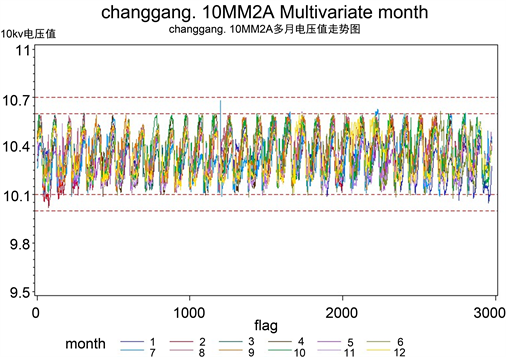Figure 1. 12 Month voltage data sequence diagram of changgang.10MM2A

4.1. 数据预处理

${V}_{miss}=\sum {V}_{i}$ (4.1)

4.2. 模型效果测量指标

$\left({V}_{i},{V}_{i+1},\cdots ,{V}_{i+n}\right)$ 为测试数据， $\left({\stackrel{˜}{V}}_{i},{\stackrel{˜}{V}}_{i+1},\cdots ,{\stackrel{˜}{V}}_{i+n}\right)$ 为根据支持向量机回归估计出的模型计算得到的预测值。

$\text{MSE}=\frac{1}{n}\underset{i=1}{\overset{n}{\sum }}{\left({V}_{i}-{\stackrel{˜}{V}}_{i}\right)}^{2}$ (4.2)

${r}^{2}=\frac{{\left(\underset{i=1}{\overset{n}{\sum }}\left({V}_{i}-{\stackrel{¯}{V}}_{i}\right)\left({\stackrel{˜}{V}}_{i}-{\stackrel{¯}{\stackrel{˜}{V}}}_{i}\right)\right)}^{2}}{\underset{i=1}{\overset{n}{\sum }}{\left({V}_{i}-{\stackrel{¯}{V}}_{i}\right)}^{2}\underset{i=1}{\overset{n}{\sum }}{\left({\stackrel{˜}{V}}_{i}-{\stackrel{¯}{\stackrel{˜}{V}}}_{i}\right)}^{2}}$ (4.3)

4.3. 模型参数确定和优化

$K\left({x}_{i},{x}_{i}\right)=\mathrm{exp}\left(-\gamma {‖{x}_{i}-{x}_{j}‖}^{2}\right)$ (4.4)

$\stackrel{¯}{\text{MSE}}=\frac{1}{K}{\sum }_{i=1}^{k}{\text{MSE}}_{i}$ (4.5)

$\stackrel{¯}{{r}^{2}}=\frac{1}{K}{\sum }_{i=1}^{k}{r}_{i}^{2}$ (4.6)Table 1. Parameter selection process of changgang.10MM2A training set ( C , γ )Table 2. Parameter selection process of changgang.10MM2A training set ε

4.4. 电压特征曲线提取Figure 2. Voltage characteristic curve of changgang.10MM2A

4.5. 特征曲线建模聚类

$V=\left({V}_{1},{V}_{2},\cdots ,{V}_{574}\right)$ (4.7)

${V}_{n}=\left({V}_{n,1},{V}_{n,2},\cdots ,{V}_{n,574}\right)$ (4.8)

$d\left({V}_{m},{V}_{n}\right)=\sqrt{\underset{i=1}{\overset{96}{\sum }}{\left({V}_{m,i}-{V}_{n,i}\right)}^{2}}$ (4.9)

$\stackrel{˜}{d}\left({V}_{m},{V}_{n}\right)=\frac{\sqrt{\underset{i=1}{\overset{96}{\sum }}{\left({V}_{m,i}-{V}_{n,i}\right)}^{2}}}{\sqrt{\underset{i=1}{\overset{96}{\sum }}{V}_{m,i}^{2}}\sqrt{\underset{i=1}{\overset{96}{\sum }}{V}_{n,i}^{2}}}$ (4.10)Table 3. Data of clustering results

5. 聚类结果及分析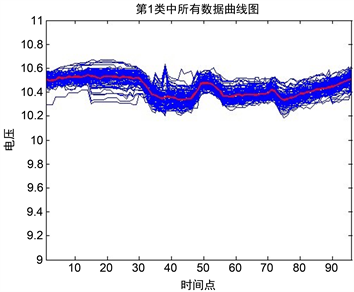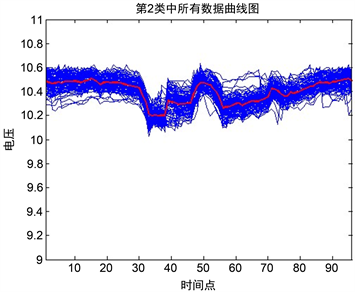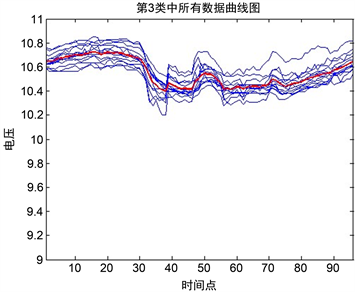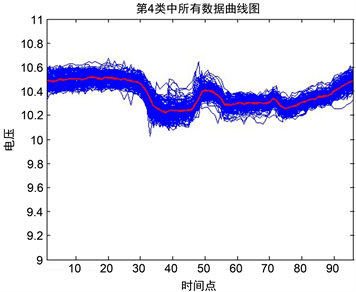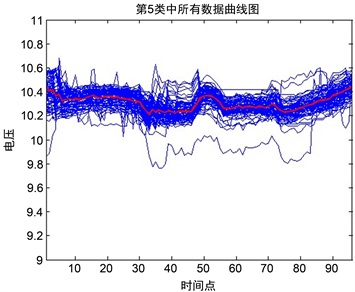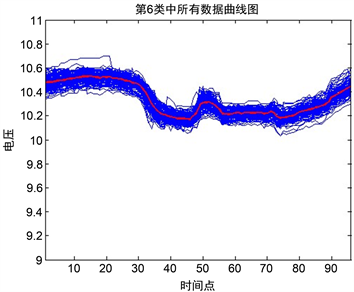Figure 3. Results of K-means clustering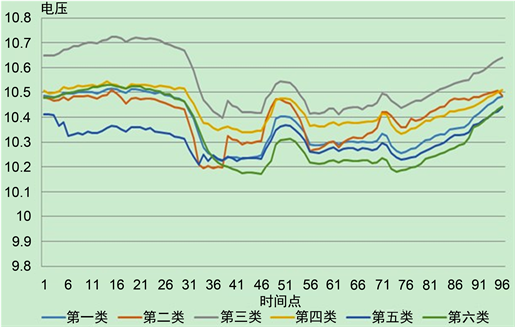Figure 4. Characteristic curve of main transformer cluster center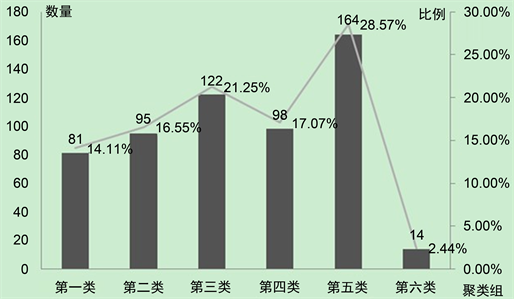Figure 5. Quantitative distribution of clustering characteristic curves

6. 结论

 邱桃荣. 变电站电压数据采集与管理系统[J]. 计算机应用, 2002(8): 114-116.

 王志勇, 曹一家. 电力客户负荷模式分析[J]. 电力系统及其自动化学报, 2007(3): 62-65.

 仲伟宽. 模糊聚类方法在用户负荷曲线分析中的应用[J]. 华东电力, 2007(8): 97-100.

 冯璐, 王成文, 申晓留, 等. 基于数据挖掘的供电企业客户关系管理系统研究与设计[J]. 电力信息化. 2007(7): 86-89.

 Vapnik, V.N. (2000) The Nature of Statistical Learning Theory. Tsinghua University Press, Beijing.
https://doi.org/10.1007/978-1-4757-3264-1

 Macqueen, J.B. (1967) Some Methods for Classication and Analysis of Multivariate Observations. In: Proceedings of the Fifth Symposium on Math, Statistics, and Probability, University of California Press, Berkeley, 281-297.

 林雄, 邢诒杏. 模糊聚类方法在负荷曲线分析中的应用[J]. 信息技术, 2008(2): 94-96.

 林智仁等. LIBSVM—A Library for Support Vector Machines [Z].

 王炜, 郭小明, 王淑艳, 刘丽琴. 关于核函数选取的方法[J]. 辽宁师范大学学报(自然科学版), 2008(1): 1-4.

 Roli, F. and Fumera, G. (2001) Support Vector Machines for Remote-Sensing Image Classification. In: Image and Signal Processing for Remote Sensing, International Society for Optics and Photonics, 160-166.
https://doi.org/10.1117/12.413892

Top